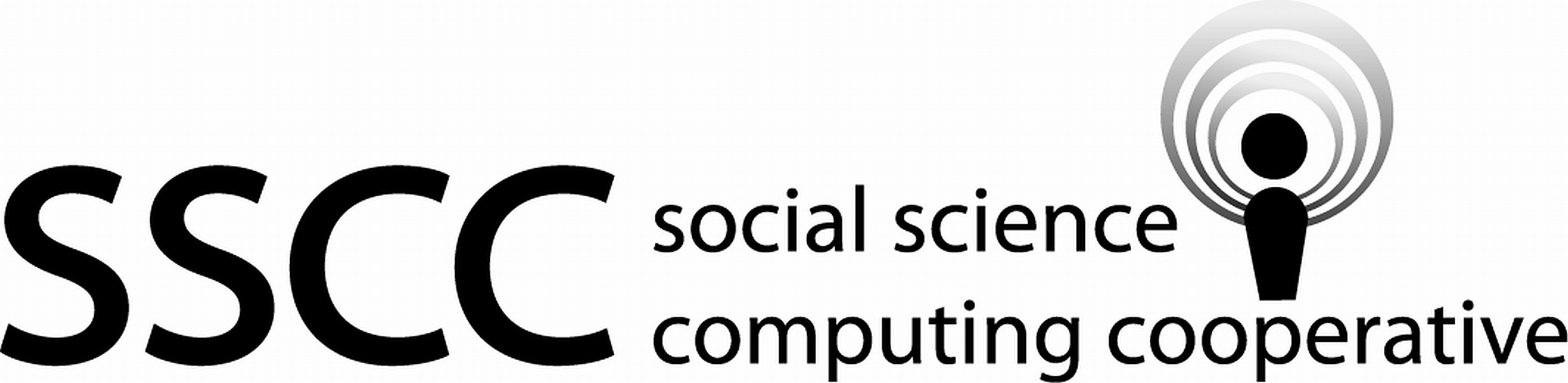Supporting Statistical Analysis for Research

## 2.8 Subsetting rows

The tidyverse provides several functions to select rows from a tibble.

• filter() selects rows using a Boolean condition.

• sample_n() and sample_frac() take a random sample of the rows.

• slice() selects row by numeric position.

### 2.8.1 Examples

1. Create test and training data frames using filter().

set.seed(145705)

cps <-
cps %>%
mutate(
split = ifelse(runif(n()) > .75, "test", "train")
)
cps_train <-
cps %>%
filter(split == "train")
cps_test <-
cps %>%
filter(split == "test")

dim(cps)    
 15992    11
dim(cps_train)    
 11902    11
dim(cps_test)    
 4090   11
2. Create test and training data frames using slice().

set.seed(145705)
test_indx <- which(runif(nrow(cps)) > .75)
train_ind <- setdiff(1:nrow(cps), test_indx)

cps_train <-
cps %>%
slice(train_ind)
cps_test <-
cps %>%
slice(test_indx)

dim(cps)    
 15992    11
dim(cps_train)    
 11902    11
dim(cps_test)    
 4090   11